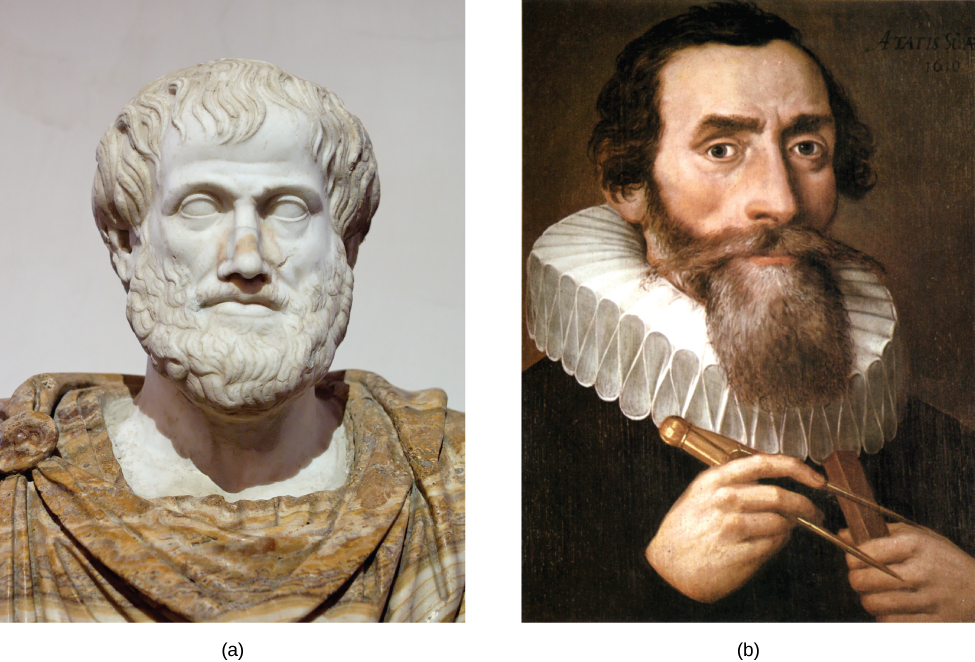# 79 Introduction to Analytic GeometryFigure 1. (a) Greek philosopher Aristotle (384–322 BCE) (b) German mathematician and astronomer Johannes Kepler (1571–1630)

The Greek mathematician Menaechmus (c. 380–c. 320 BCE) is generally credited with discovering the shapes formed by the intersection of a plane and a right circular cone. Depending on how he tilted the plane when it intersected the cone, he formed different shapes at the intersection–beautiful shapes with near-perfect symmetry.

It was also said that Aristotle may have had an intuitive understanding of these shapes, as he observed the orbit of the planet to be circular. He presumed that the planets moved in circular orbits around Earth, and for nearly 2000 years this was the commonly held belief.

It was not until the Renaissance movement that Johannes Kepler noticed that the orbits of the planet were not circular in nature. His published law of planetary motion in the 1600s changed our view of the solar system forever. He claimed that the sun was at one end of the orbits, and the planets revolved around the sun in an oval-shaped path.

In this chapter, we will investigate the two-dimensional figures that are formed when a right circular cone is intersected by a plane. We will begin by studying each of three figures created in this manner. We will develop defining equations for each figure and then learn how to use these equations to solve a variety of problems.